# Concept of Pie Graph (Or a Circle-graph)

#### definition

Pie graph(Or a Circle-graph): A pie-graph is used to compare parts of a whole. A circle graph shows the relationship between a whole and its part. Here, the whole circle is divided into sectors. The size of each sector is proportional to the activity or information it represents.

# Pie graph(Or a Circle-graph):

• A pie-graph is used to compare parts of a whole.

• Data can also be presented using a circle graph or pie chart. A circle graph shows the relationship between a whole and its part. Here, the whole circle is divided into sectors. The size of each sector is proportional to the activity or information it represents.

The following pie graph shows the percentage of viewers watching different types of TV channels.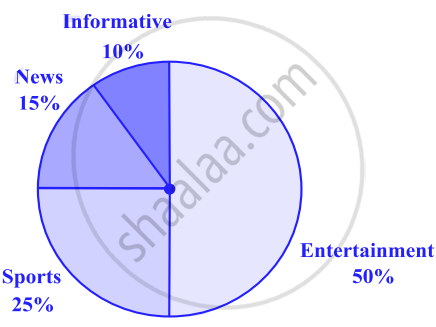# Drawing pie charts:

1) The favourite flavours of ice-creams for students of a school are given in percentages as follows.

### Percentage of studentsPreferring the flavours

Chocolate

50%

Vanilla

25%

Other flavours

25%

Let us represent this data in a pie chart.

The total angle at the centre of a circle is 360°. The central angle of the sectors will be a fraction of 360°. We make a table to find the central angle of the sectors.

### Fraction of 360°

Chocolate 50% 50/100 = 1/2 1/2  "of"  360° =180°
Vanilla 25% 25/100 = 1/4 1/4  "of"  360° = 90°
Other flavours 25% 25/100 = 1/4 1/4  "of"  360° = 90°

1. Draw a circle with any convenient radius. Mark its centre (O) and a radius (OA).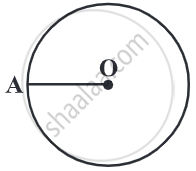2. The angle of the sector for chocolate is 180°. Use the protractor to draw ∠AOB = 180°.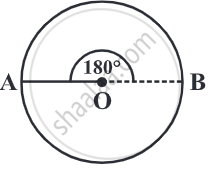3. Continue marking the remaining sectors.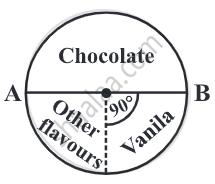#### Example

On a particular day, the sales (in rupees) of different items of a baker’s shop are given below.
 ordinary bread 320 fruit bread 80 cakes and pastries 160 biscuits 120 others 40 Total 720
Draw a pie chart for this data.
We find the central angle of each sector. Here the total sale = Rs. 720. We thus have this table.
 Item Sales (In Rs.) In Fraction Central Angle Ordinary Bread 320 320/720 = 4/9 4/9xx360° = 160° Fruit Bread 80 80/720 = 1/9 1/9xx360° = 40° Cakes and pastries 160 160/720 = 2/9 2/9xx360° = 80° Biscuits 120 120/720 = 1/6 1/6xx360° = 60° Others 40 40/720 = 1/18 1/18xx360° = 20°
Now, we make the pie chart: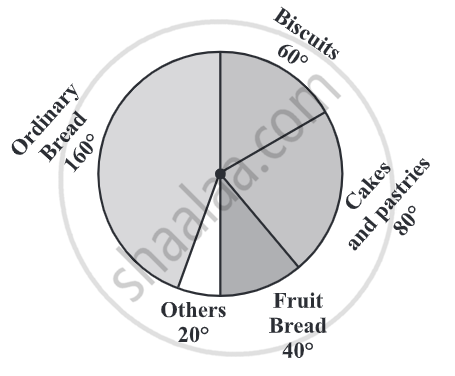If you would like to contribute notes or other learning material, please submit them using the button below.

#### Video Tutorials

We have provided more than 1 series of video tutorials for some topics to help you get a better understanding of the topic.

Series 1

Series 2

### Shaalaa.com

Drawing Pie Charts [00:08:52]
S
0%